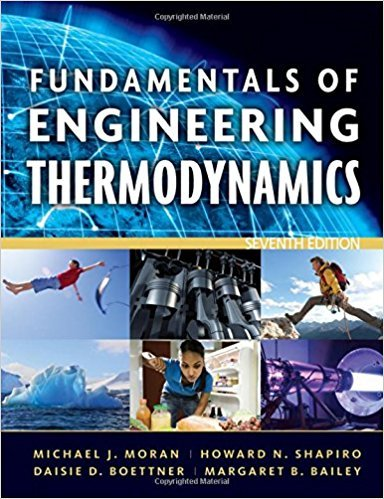×
Get Full Access to Fundamentals Of Engineering Thermodynamics - 7 Edition - Chapter 1 - Problem 36p
Get Full Access to Fundamentals Of Engineering Thermodynamics - 7 Edition - Chapter 1 - Problem 36p

×

# Using Specific Volume, Volume, and PressureWater flowsISBN: 9780470495902 50

## Solution for problem 36P Chapter 1

Fundamentals of Engineering Thermodynamics | 7th Edition

• Textbook Solutions
• 2901 Step-by-step solutions solved by professors and subject experts
• Get 24/7 help from StudySoup virtual teaching assistantsFundamentals of Engineering Thermodynamics | 7th Edition

4 5 1 391 Reviews
27
1
Problem 36P

Problem 36P

Using Specific Volume, Volume, and Pressure

Water flows through a Venturi meter, as shown in Fig. P1.36. The pressure of the water in the pipe supports columns of water that differ in height by 10 in. Determine the difference in pressure between points a and b, in lbf/in.2 Does the pressure increase or decrease in the direction of flow? The atmospheric pressure is 14.7 lbf/in.2, the specific volume of water is 0.01604 ft3/lb, and the acceleration of gravity is g = 32.0 ft/s2.Fig. P1.36

Step-by-Step Solution:

## Step 1 of 2

We have to calculate the pressure difference for the given height difference.

Step 2 of 2

##### ISBN: 9780470495902

Since the solution to 36P from 1 chapter was answered, more than 387 students have viewed the full step-by-step answer. This full solution covers the following key subjects: pressure, Water, Volume, lbf, specific. This expansive textbook survival guide covers 14 chapters, and 1501 solutions. The full step-by-step solution to problem: 36P from chapter: 1 was answered by , our top Engineering and Tech solution expert on 07/20/17, 09:01AM. Fundamentals of Engineering Thermodynamics was written by and is associated to the ISBN: 9780470495902. The answer to “Using Specific Volume, Volume, and PressureWater flows through a Venturi meter, as shown in Fig. P1.36. The pressure of the water in the pipe supports columns of water that differ in height by 10 in. Determine the difference in pressure between points a and b, in lbf/in.2 Does the pressure increase or decrease in the direction of flow? The atmospheric pressure is 14.7 lbf/in.2, the specific volume of water is 0.01604 ft3/lb, and the acceleration of gravity is g = 32.0 ft/s2. Fig. P1.36” is broken down into a number of easy to follow steps, and 84 words. This textbook survival guide was created for the textbook: Fundamentals of Engineering Thermodynamics, edition: 7.

Unlock Textbook Solution